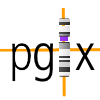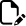## Clustering

The Progenetix CNV visualization includes some default clustering for sample CNV profiles and collation CNV frequencies.

The resource uses a standard CNV binning model with (in GRCh38) 3102 intervals of 1MB default size. For the clustering of both status and frequency values, gain and loss intervals are treated independently, resulting in 6204 values per sample (or collation) for the generation of a clustering matrix:

• for sample clustering, the cluster matrix for each sample (line) consists of the fraction of each 1Mb bin covered by a gain (resp. loss) in this sample
• this results for CNVs larger than 1Mb in several bins with `1.000`, and then the edge bins with values below 1
• for CNV frequencies (e.g. comparing results for different cancer types) the values are the percents of samples having a CNV of the given type per 1Mb bin

On the website sample and/or collation clustering is involved automatically on the “Data Visualization” page which can be accessed from the search results. Clustering as well as CNV visualization are performed through the PGX Perl library, utilizing the `Algorithm::Cluster` interface to the C cluster library with default parameters:

• `m`: pairwise complete-linkage clustering
• `e`: Euclidean distance

For visualization, while clustering is performed on the matrix with separate values for gains and losses, the plotting is then performed on the original data:

• samples are ordered as determined from the clustering, but then the original non-binned CNV segments are plotted along the genome
• this results in the natural sequence of gain and loss segments, not in a separate display
• for CNV frequencies, a mixed color is generated fro the combined gain and loss values for each 1Mb bin
• technically, each of the red, green, blue channels of the RGB values for the gain and loss frequencies the channel values for DUP and DEL are added, normalized for the overall maximum CNV frequency in the data
``````for my \$i (0..2) {
\$dupRGB->[\$i] = int(\$dupRGB->[\$i] * \$dupF / \$maxF);
\$delRGB->[\$i] = int(\$delRGB->[\$i] * \$delF / \$maxF);
if ((\$dupRGB->[\$i] + \$delRGB->[\$i]) < 255) {
\$RGB[\$i] = \$dupRGB->[\$i] + \$delRGB->[\$i] }
else {
\$RGB[\$i] = 255 }
}
``````

Clustering can be omitted by setting `Cluster Tree Width` to `0`.